# Simple-iteration method

(diff) ← Older revision | Latest revision (diff) | Newer revision → (diff)

A method for approximately solving a system of linear algebraic equationsthat can be transformed to the form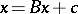and whose solution is looked for as the limit of a sequence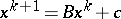,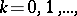where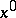is an initial approximation. In order that the simple-iteration method converges for any initial approximationit is necessary and sufficient that all eigenvalues ofare less than one in modulus; it is sufficient that some norm ofis less than one. If in some norm, compatible with the norm of a vector,satisfies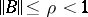, then the simple-iteration method converges at the rate of a geometric series and the estimate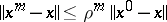holds for its error.

In the case of a cubic, octahedral or spherical vector norm, the conditionis fulfilled if

1)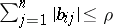,;

2)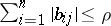,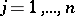;

3)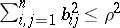.

The simplest version of the method corresponds to the case when one takes, whereis the identity matrix, for. If all diagonal entries ofare non-zero, then, choosing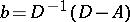and, whereis the diagonal matrix with as diagonal entries those of, one obtains the Jacobi method or the method of simultaneous displacement.

A particular case of the simple-iteration method is the method withand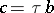, whereis an iteration parameter, chosen from the condition that the norm ofis minimal with respect to. If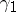and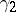are the minimal and maximal eigenvalues of a symmetric positive-definite matrixand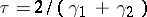, then one has for the matrixin the spherical norm the estimate, with.

For a system of non-linear algebraic equations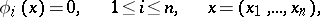the simple-iteration method has the form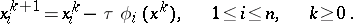The problem of choosing the iteration parameteris solved in dependence on the differentiability properties of the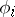. Often it is subjected to the requirement that the method converges locally in a neighbourhood of a solution.

How to Cite This Entry:
Simple-iteration method. Encyclopedia of Mathematics. URL: http://encyclopediaofmath.org/index.php?title=Simple-iteration_method&oldid=16747
This article was adapted from an original article by E.S. Nikolaev (originator), which appeared in Encyclopedia of Mathematics - ISBN 1402006098. See original article